# Circles - Olympiad Level MCQ, Class 10 Mathematics

## 25 Questions MCQ Test Olympiad Preparation for Class 10 | Circles - Olympiad Level MCQ, Class 10 Mathematics

Description
Attempt Circles - Olympiad Level MCQ, Class 10 Mathematics | 25 questions in 25 minutes | Mock test for Class 10 preparation | Free important questions MCQ to study Olympiad Preparation for Class 10 for Class 10 Exam | Download free PDF with solutions
QUESTION: 1

Solution:
QUESTION: 2

Solution:
QUESTION: 3

### In the given figure, AP = 3 cm, BA = 5 cm and CP = 2 cm. Find CD :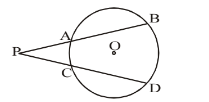Solution:
QUESTION: 4

In the given figure, tangent PT = 5 cm, PA = 4 cm, find AB :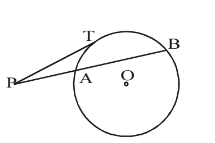Solution:
QUESTION: 5

Two circles of radii 13 cm and 5 cm touch internally each other. Find the distance between their centres :

Solution:
QUESTION: 6

Three circles touch each other externally. The distance between their centre is 5 cm, 6 cm and 7 cm. Find the radii of the circles :

Solution:
QUESTION: 7

If AB is a chord of a circle, P and Q are two points on the circle different from A and B, then:

Solution:
QUESTION: 8

In the given figure, CD is a direct common tangent to two circles intersecting each other at A and B, then: ∠CAD + ∠CBD = ?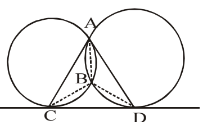Solution:
QUESTION: 9

In a circle of radius 5 cm, AB and AC are the two chords such that AB = AC = 6 cm. Find the length of the chord BC.

Solution:
QUESTION: 10

In a circle of radius 17 cm, two parallel chords are drawn on opposite sides of a diameter. The distance between the chords is 23 cm. If the length of one chord is 16 cm, then the length of the other is :

Solution:
QUESTION: 11

If two circles are such that the centre of one lies on the circumference of the other, then the ratio of the common chord of two circles to the radius of any of the circles is :

Solution:
QUESTION: 12

Two circles touch each other internally. Their radii are 2 cm and 3 cm. The biggest chord of the other circle which is outside the inner circle, is of length :

Solution:
QUESTION: 13

Through any given set of four points P,Q, R, S it is possible to draw :

Solution:
QUESTION: 14

What is the probability, that a number selected from 1, 2, 3, …, 25, is a prime number, when each of the numbers is equally likely to be selected.

Solution:

S = { 1, 2, 3, ---- , 25} |S|= 25 And E = { 2, 3, 5, 7, 11, 13, 17, 19, 23 } |E|= 9 Hence P(E) = |E| / |S| = 9 / 25

QUESTION: 15

The number of common tangents that can be drawn to two given circles is at the most :

Solution:

When two circles touch each other externally, 3 common tangents can be drawn to the circles.

When two circle neither touch nor intersect and one lies outside the other, then 4 common tangents can be drawn.

QUESTION: 16

ABC is a right angled triangle AB = 3 cm, BC = 5 cm and AC = 4 cm, then the inradius of the circle is :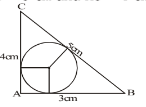Solution:
QUESTION: 17

In the given figure O is the centre of the circle and ∠BAC = 25°, then the value of ∠ADB is :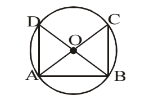Solution:
QUESTION: 18

In the given circle O is the centre of the circle and AD, AE are the two tangents. BC is also a tangent, then :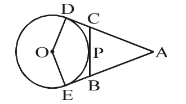Solution:
QUESTION: 19

In a circle O is the centre and ∠COD is right angle. AC = BD and CD is the tangent at P. What is the value of AC + CP, if the radius of the circle is 1 metre?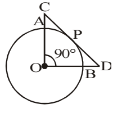Solution:
QUESTION: 20

In a triangle ABC, O is the centre of incircle PQR, ∠BAC = 65°, ∠BCA = 75°, find ∠ROQ :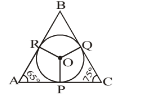Solution:
QUESTION: 21

In the adjoining figure O is the centre of the circle. ∠AOD = 120°. If the radius of the circle be 'r', then find the sum of the areas of quadrilaterals AODP and OBQC :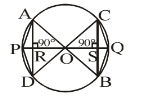Solution:
QUESTION: 22

There are two circles each with radius 5 cm. Tangent AB is 26 cm. The length of tangent CD is :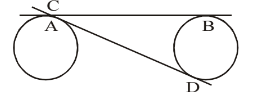Solution:
QUESTION: 23

In the adjoining figure O is the centre of the circle and AB is the diameter. Tangent PQ touches the circle at D. ∠BDQ = 48°. Find the ratio of ∠DBA : ∠DCB :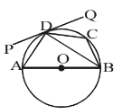Solution:
QUESTION: 24

In the given diagram an incircle DEF is circumscribed by the right angled triangle in which AF = 6 cm and EC = 15 cm. Find the difference between CD and BD :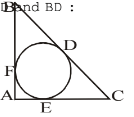Solution:
QUESTION: 25

In the adjoining figure 'O' is the centre of circle, ∠CAO = 25° and ∠CBO = 35°. What is the value of ∠AOB?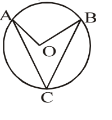Solution:Use Code STAYHOME200 and get INR 200 additional OFF Use Coupon Code